##### What are Operators?

An operator is a symbol that tells the compiler to perform specific mathematical, logical calculations or some other special operations.

In C++, we can make operators work for user-defined classes. This means C++ has the ability to provide the operators with a special meaning for a data type, this ability is known as operator overloading. For example, we can overload the + operator in a class like String so that we can concatenate two strings by just using +.

Using operator overloading in C++, we can specify more than one meaning for an operator in one scope. The purpose of operator overloading is to provide a special meaning of an operator for a user-defined data type.

To overload an operator in C++, we use a special operator function. We define the function inside the class or structure whose objects/variables we want the overloaded operator to work with. The Syntax for Operator Overloading as shown in the below image.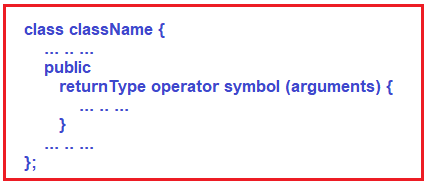Here,

1. returnType is the return type of the function.
2. the operator is a keyword.
3. the symbol is the operator that we want to overload. Like: +, <, -, ++, etc.
4. arguments are the arguments passed to the function.

We have operators for performing addition (+), multiplication (*), subtraction (-), conversion (“()”) operation, increment and decrement operator (++, –), new Operator, delete operator, and so on. That means to perform various kinds of things there are operators available in C++. And these operators are meant for some specific data types.The above image shows some built-in operators and these operators are operates on built-in data types or primitive data types available in C++. Like additions can be performed on integer, float, and so on. If we are defining our own data type like if we are writing a class Matrix.

class Matrix {
——–
}

Then can we write the operator for adding two matrices and store the result in another object of type matrix (“C = A + B”)? So, can we overload the ‘+’ operator for the matrix class? Yes, the ‘+’ operator can be overloaded for the matrix class.

So, for our own data type i.e. user-defined data types, we can overload the ‘+’ operator. There are various operators that you can overload in C++. So let us learn how to overload these operators.

Let us take an example of a complex number to understand Operator Overloading in C++. In mathematics, we have a complex number that is written in the form of a + ib as shown in the below image.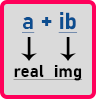Here a is the real part and ib is the imaginary part. What is imaginary? Here, ‘i’ is the square root of -1.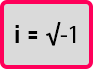The square root of minus one is undefined. So, we call it imaginary (‘i’). Anything multiplied by an imaginary number becomes an imaginary number. Here, a is an integer or float number and ib is imaginary. If we have two complex numbers then we can add them by adding their real part and imaginary part separately. If we have 3 + 7i and 5 + 2i then after addition we will get 8 + 9i. We got this as the addition of two complex numbers.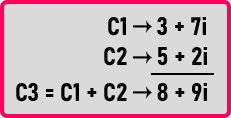So yes, we can perform addition on two complex numbers. The same thing we want to achieve programmatically then we want to have + operator overloaded. So let us write a class for a complex number as shown below and see how we can overload the + operator.

```class Complex{
private:
int real;
int img;
public:
Complex(int r = 0, int i = 0){
real = r;
img = i;
}
};
```

Here, we have created a class Complex. Inside the Complex class, we have created two integer-type private data members that are real and img. Then we have created a parameterized constructor as public. We can pass the two integer values as parameters into the constructor and the constructor will assign those integer values to real and img private data members of the class.

We have also provided some default values to the constructor arguments so that if the user doesn’t pass any values, then the constructor will automatically assign 0 to real and img data members. This constructor will work as a parameterized constructor as well as a non-parameterized constructor.

Now let us overload + operator. For learning operator overloading we have to learn two things. First, how to write a function, and second, what should be the signature of a function. Signature of a function we will show you afterward, first, let us see how to write a function.

class Complex{
…
Complex temp;
temp.real = real + x.real;
temp.img = img + x.img;
return temp;
}
};

Here inside the Complex class, we have written add function of the return type of this function is Complex. This function will add the real and img values of two Complex objects. Now let us write the main function as follows:

int main(){
Complex C1 (3, 7);
Complex C2 (5, 2);
Complex C3;
}

Here inside the main function, we have created two objects C1 and C2 of class Complex. C1 will have the values of 3 and 7 and C2 will have the values of 5 and 2. Then we have created another object of Complex that is C3. We have not passed any parameter so the constructor will assign 0 value to real and img variables of C3. Then with the help of the C1 object, we are calling add function and pass the C2 object as a parameter. And storing the result in object C3. So C1 object will perform addition with C2’s data members.

Suppose there are two-person X and Y. X has some money and Y also has some money. Now they want to add their money. So, who will add? X also knows how to add and Y also knows how to add money. If X wants to add then X will take money from Y as a parameter and add Y’s money with his money. Or else Y will take X money as a parameter and add X’s money with his money. So, either X can add the money or Y can add the money.

So, in the same way, C1 will add by taking C2 as an argument or C2 can also add by taking C1 as an argument.

##### How add function is working?

Now let us understand how add function is working.
In the above statement, the add function will be called upon the C1 object and C2 passes as a parameter.
Complex temp;
Then inside the add function, a temporary variable of type Complex that is temp will be created. Then,
temp.real = real + x.real;
This statement will store the addition of values of C1’s real and C2’s real in temp’s real. Here just real means C1’s real and x.real means C2’s real because C2 is x here in the function. Then,
temp.img = img + x.img;
This statement will store the addition of values of C1’s img and C2’s img in temp’s img. Here just img means C1’s img and x.img means C2’s img.
return temp;
Then we have returned the temp object from the function. We can understand the above statement with the help of the below diagram.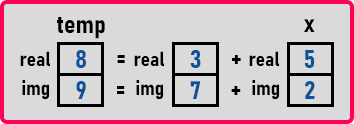This diagram represents that we have stored the result of the addition of C1 and C2 into the temp variable which is of the Complex type. the temp will be returned by the add function. So inside the main function, we just store the temp data in the C3 object. For a better understanding, please have a look at the below image.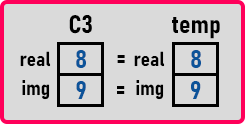So, this is how addition is done. So, this logic is important. How to write a function is important. Now we are modifying objects as,

Here, the add function will be called upon the C2 object and C1 will be passed as a parameter. Either add function can be called upon the C1 object or it can be called upon the C2 object.

##### Complete Example to add two Complex Numbers in C++:
```#include<iostream>
using namespace std;

class Complex
{
private:
int real;
int img;
public:
Complex (int r = 0, int i = 0)
{
real = r;
img = i;
}
{
Complex temp;
temp.real = real + x.real;
temp.img = img + x.img;
return temp;
}
void Display() {
cout << real << "+i" << img <<endl;
}
};

int main()
{
Complex C1 (3, 7);
C1.Display();
Complex C2 (5, 2);
C2.Display();
Complex C3;
C3.Display();
}
```
###### Output: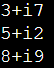Understanding logic is the most important thing. So, we have finished it. Now let us see how to make it as operator overloading. Now we want to convert add function into an operator. So, instead of writing C3 = C2.add(C1), we want to write C3 = C2 + C1; So, for writing like this, we have to modify the function signature as follows:

Complex operator + (Complex x){
…
}

Here, we just replace the add word with operator +. Everything inside the function will remain the same as the previous one. With the above changes in place, now the + operator is overloaded for class Complex. This is Operator Overloading in C++. So instead of writing dot, you can just write ‘+’ to get the addition of two Complex objects. Now let us look at the complete program for operator overloading in C++.

```#include<iostream>
using namespace std;

class Complex
{
private:
int real;
int img;
public:
Complex (int r = 0, int i = 0)
{
real = r;
img = i;
}
Complex operator + (Complex x)
{
Complex temp;
temp.real = real + x.real;
temp.img = img + x.img;
return temp;
}
void Display() {
cout << real << "+i" << img <<endl;
}
};

int main()
{
Complex C1 (3, 7);
C1.Display();
Complex C2 (5, 2);
C2.Display();
Complex C3;
C3 = C1+C2;
C3.Display();
}
```
###### Output:Note: In C++, the Operator functions are the same as normal functions. The only difference is that the name of an operator function is always the operator keyword followed by the symbol of the operator and operator functions are called when the corresponding operator is used.

##### Can we overload all operators in C++?

In C++, almost all operators can be overloaded except a few. Following is the list of operators that cannot be overloaded in C++.
. (dot)
::
?:
sizeof

Please check the below article on why we can not overload the above operators.

We already know that the unary operators operate only on one operand. The increment operator ++ and decrement operator — are examples of unary operators. Let us understand this with an example. In the below example we will overload the unary ++ operator. In the below example, when we use ++count1; when this statement is executed, the void operator ++ () function will be called and this function will increases the value attribute for the object count1 by 1.

```#include <iostream>
using namespace std;

class Count{
private:
int value;

public:
Count(int num = 0)
{
value = num;
}

// Overload ++ when used as prefix
void operator ++ (){
++value;
}

void Display(){
cout << "Count: " << value << endl;
}
};

int main() {
Count count1(10);
count1.Display();
// Call the "void operator ++ ()" function
++count1;

count1.Display();
return 0;
}
```
###### Output: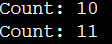The above example works only when ++ is used as a prefix. To make ++ work as a postfix we need to use the following syntax.

void operator ++ (int) {
// code
}

Notice that int inside the parentheses. It’s the syntax used for using unary operators as postfix; it’s not a function parameter.

##### Example to Overload ++ when used as Prefix and Postfix in C++:
```#include <iostream>
using namespace std;

class Count{
private:
int value;

public:
Count(int num = 0)
{
value = num;
}

// Overload ++ when used as prefix
void operator ++ (){
++value;
}

// Overload ++ when used as postfix
void operator ++ (int){
++value;
}

void Display(){
cout << "Count: " << value << endl;
}
};

int main() {
Count count1(10);
count1.Display();
// Call the "void operator ++ ()" function
count1++;
count1.Display();
++count1;
count1.Display();
return 0;
}
```
###### Output: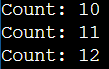The above Unary Operator Example works fine when ++ is used as both prefix and postfix. However, it doesn’t work if we try to do something like the below:

Count count1, result;
// Error
result = ++count1;

This is because the return type of operator ++ function is void. We can solve this problem by making Count the return type of the operator function as follows:

Count operator ++ () {
// code
}

Count operator ++ (int) {
// code
}

##### Example to Return Value from Operator Function (++ Operator) in C++:
```#include <iostream>
using namespace std;

class Count{
private:
int value;

public:
Count(int num = 0)
{
value = num;
}

// Overload ++ when used as prefix
Count operator ++ (){
Count temp;
// Here, value is the value attribute of the calling object
temp.value = ++value;
return temp;
}

// Overload ++ when used as postfix
Count operator ++ (int){
Count temp;
// Here, value is the value attribute of the calling object
temp.value = value++;
return temp;
}

void Display(){
cout << "Count: " << value << endl;
}
};

int main() {
Count count1(10), result;
result = ++count1;
result.Display();

result = count1++;
result.Display();
return 0;
}
```
###### Output: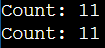1.